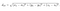# Data monitoring system in Python

## Introduction

For analytics purposes we use different data visualization tools like Google DataStudio and Tableau. They fetch data from SQL views deployed on Postgres and show beautiful graphs.

## Problem statement

Sometimes sudden spikes appear on the graphs. In most cases, they are caused by an issue on backend or client side like a bug in purchase handler or recent deployment that went wrong. The problem will be fixed in a while but the data is already affected and graphs show wrong numbers. It would be more convenient if we can receive a notification about any ‘weird’ numbers and prevent Tableau dashbords refreshment before wrong numbers appeared.

## Solution

First, we can try to compare difference between two numbers and understand whether it’s significant or not. If we calculate the percentage, we’ll find out that such way isn’t very convenient. Here is an example:

Difference in number of bypasses (1 and 3) between last two days is 200%. Let’s try to compare average value of 3 days and the last day: average number is (0+0+1)/3=0.33. Difference between average value and value from 01.04 is 900%.

As we can see, for ‘small’ numbers such a huge difference as 200% and 900% is acceptable. Let’s apply the same calculations to ‘big’ numbers:

Difference in number of registrations between 920 and 600 is 25%, difference between average value ((800+750+920)/3=823) and 690 is 16%.

Looks like the percentage itself is not representative because both 900% and 16% are acceptable. We have to come up with some additional coefficient for each kind of data.

That is where Euclidian distance comes into action. We’ll represent sets of data (table rows) as points in N-dimensional space where number of columns is equal to the number of dimensions. This is an example of two points a and b in 3-dimensional space:Formula of distance between two points a and b in 3D space

Let’s iterate through each pair of rows calculating distances between them. In our case, each row is a point in 4D space. We can either use euclidean() from SciPy or, if we don’t want to use the whole 28MB library for one function only, can write a simple implementation:

Next step is comparing one distance to the median distance:

We could introduce some multiplicator coefficient for the median value and compare the difference to coefficient*median_distance instead of just median_distance. It depends on how ‘sensitive’ your alarm should be.

That’s it. The algorithm is very simple and can be easily integrated into existing infrastructure. As one of possible solutions, you can run it in AWS Lambda and send notifications to your email or Slack channel.

## More from Viktor Savelev

Backend Developer